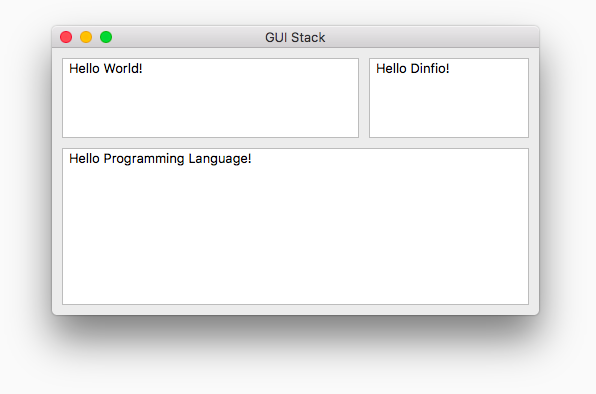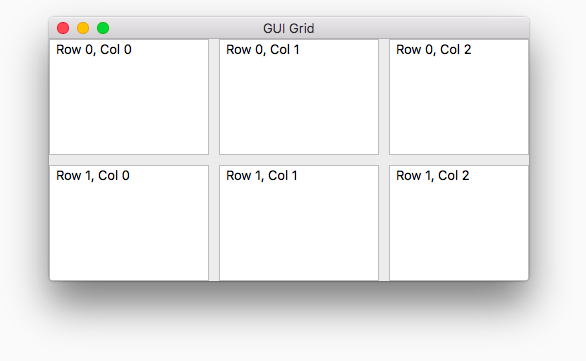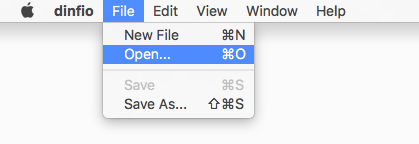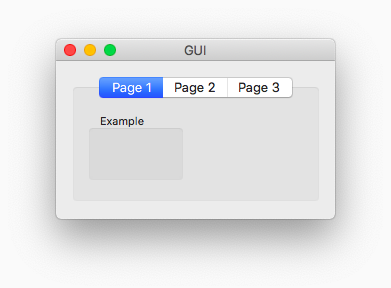### Dinfio Release Notes

###### Dinfio 3.1.06 ⋅ Release date: 2022-01-14

1. Dinfio is now open source! Please check out the repository here: https://github.com/dinfio/dinfio
2. Added default value in function parameters
``````start
something(20)       ' x = 20, y = 10 (use default value)
something(20, 40)   ' x = 20, y = 40
stop

function something(x, y = 10)
writeln("x: " & x)
writeln("y: " & y)
stop``````

3. Operators `==` and `!=` now support for array and object operands
``````a = {name: "Sarah", age: 20}
b = {name: "Sarah", age: 20}
c = a

' Dinfio 3.1.05 or earlier

x = equal(a, b)      ' false
y = equal(a, c)      ' true
z = !is_nothing(a)   ' true

' Dinfio 3.1.06

x = a == b           ' false
y = a == c           ' true
z = a != nothing     ' true``````

4. Removed insensitive case syntax for a consistent code
5. Added module `rl` to provide advanced readline functions
6. Added bitwise functions `bnot()`, `band()`, `bor()`, `bxor()`, `bls()`, and `brs()`to module `core`
7. Added function `eof()` to module `standardio`
8. Added functions `attribute_exists()`, `attribute_get()`, and `attribute_set()` to module `core`
9. Added function `fileput()` to module `fileio`
10. Added function `reopen()` to class `file` in module `fileio`
11. Added parameter `is_password` to class constructor `gui_textbox::construct()` in module `gui`
12. Fixed crash issue on non-editable `gui_combobox` when it is added to `gui_stack` or `gui_grid`

###### Dinfio 3.1.05 ⋅ Release date: 2021-07-08

1. Added module `multiprocess` to enable process-based parallelism in Dinfio
``````' This is an example how to make 100 HTTP requests in parallel

import multiprocess

start
for i, 1, 100
process("worker", [i], void(), void(), void())
endfor
stop``````
``````' worker.fio

import multiprocess
import url, json

start
response = url.get("https://httpbin.org/uuid")

if response.code == 200
data = json.decode(response.body)
writeln("Done #" & process_arguments & ": " & data.uuid)
else
writeln("Done #" & process_arguments & ": Error " & response.code)
endif
stop``````

2. Added class `gui_stack` and `gui_grid` to module `gui`
``````' GUI Stack example

import gui

start
window = gui_window("GUI Stack", 480, 300)
stack = gui_stack(vertical, window)
panel = gui_panel(window)
hstack = gui_stack(horizontal, panel)

text1 = gui_textarea("Hello World!", panel)
text2 = gui_textarea("Hello Dinfio!", panel)
text3 = gui_textarea("Hello Programming Language!", window)

window.show()
stop``````
``````Output:``````
``````' GUI Grid example

import gui

start
window = gui_window("GUI Grid", 480, 300)
grid = gui_grid(2, 3, window, 10, 10, true)

text1 = gui_textarea("Row 0, Col 0", window)
text2 = gui_textarea("Row 0, Col 1", window)
text3 = gui_textarea("Row 0, Col 2", window)
text4 = gui_textarea("Row 1, Col 0", window)
text5 = gui_textarea("Row 1, Col 1", window)
text6 = gui_textarea("Row 1, Col 2", window)

window.show()
stop``````
``````Output:``````

3. Added class `gui_menubar` and `gui_menu` to module `gui`
``````' GUI Menu example

import gui

start
window = gui_window("GUI Menu", 450, 300)

window.show()
stop``````
``````Output:``````

4. Added class `dialog` to module `gui` to show open file, save file, and choose directory dialog
5. Added class `clipboard` to module `gui`
6. Added function `setfullscreen()` and `isfullscreen()` to class `gui_window` in module `gui`
7. Added parameter `resizable`, `minimisable`, and `closable` to `gui_window` constructor
8. Added parameter `wait` to function `execute()`
9. Added function `register_event_loop()` to register user-defined event loop
10. Fixed issue on Unicode characters that do not appear on all GUI objects
11. Fixed issue on assignment cache:
``````' Issue on version <= 3.1.04

for i, 1, 10
x = i
writeln(x)   ' x is always be false after the first iteration. It should be 2, 3, 4, 5, ...
x = false
endfor``````

12. Module `asynchronous` is now deprecated, please use module `multiprocess` instead
13. Function `sleep()` and `sleep_s()` are now moved to module `time`, which was previously in `asynchronous`

###### Dinfio 3.1.04 ⋅ Release date: 2021-04-26

1. Introduced Dima and Dinfio Modules repository
2. Dinfio is now available for Raspberry Pi and Docker!
3. Dinfio 3.1.04 is now with up to 5x faster performance and 20% less memory usage than Dinfio 3.1.03
4. Added function parameter type `ref`, class `ref`, function `call()`, and function `eval()` to store function address:
``````do_something(write())   ' Address of write() will be stored to parameter f

function do_something(ref: f)
call(f, "Hello")
stop

' Another example

f = ref(some_func())   ' Address of some_func() will be stored to variable f
call(f, 4, 10, 2)

function some_func(x, y, z)
writeln(x ^ 2 + y + z)
stop``````

5. Added module `zip` to manage ZIP archives. See the module reference for more information
6. Added module `time`. See the module reference for more information
7. Added function `var_exists()` to check whether a variable is declared or not
8. Added function `error()` to print an error message and exit from the program
9. Added functions `listdirs()` and `listfiles()` in module `fileio`
10. Added class `gui_imagebox` in module `gui`
11. Added drawing functions to class `gui_panel` in module `gui`
12. Fixed issue on `file::readstring()` and `file::writebyte()` in module `fileio`
13. Fixed frozen menubar issue on macOS Catalina and later in module `gui`

###### Dinfio 3.1.03 ⋅ Release date: 2021-01-23

1. Added module `url` to make HTTP requests. See the module reference for more information
2. Added module `json` to encode and decode JSON string. See the module reference for more information
3. Added Interactive Mode feature (REPL—Read, Eval, Print, Loop). Just type `dinfio` in your Terminal/Command Prompt:
``\$ dinfio``
``````Welcome to Dinfio Interactive Mode
Version: 3.1.03 (Linux x86_64)

Module math, string, and fileio are already imported.
Type "help" to get help.

>> 6 + 2 * 4
14
>> █``````

4. Now you can import other `fio` files in your program:
``````import other
import helper

' Call function from file other.fio

do_something()

' Constant from file other.fio

writer(some_data)

' Call function from file helper.fio

do_again(20)``````

File `other.fio`:
``````' All constants and global variables must be declared inside the init function,
' because all of codes at the outside of functions and classes will not be executed.
'
' Function name must be <unique_name>::init, you can use <filename>::init

function other::init()
global some_data = {
x: 10,
y: 20,
z: 30
}
stop

function do_something()
some_data.x = 40
stop``````

File `helper.fio`:
``````function do_again(x)
writeln(x ^ 2)
stop``````

5. Fixed issue on keyword `return`. The keyword should halt the execution of the rest codes below the keyword, instead of continue the execution.

###### Dinfio 3.1.02 ⋅ Release date: 2020-12-15

1. Added constant `arguments` to get command line arguments. For example (file name: `hello.fio`):
``writer(arguments)``

Run it:
``\$ dinfio hello.fio this is arguments``

Output:
``````array(
 = "hello.fio"
 = "this"
 = "is"
 = "arguments"
)``````

2. Added support for `# comment` as well as hashbang feature. For example (file name: `hello.fio`):
``````#!/usr/bin/env dinfio

' This is a comment
# This is also a comment

writeln("Hello, world!")``````

Run it without `dinfio` prefix (don't forget to enable execute permission `chmod +x hello.fio`):
``\$ ./hello.fio``

Output:
``Hello, world!``

``````[a, b, c] = [10, 20, 30]          ' Multiple assignment
[x, y] = 23                       ' Multiple assignment with one value
[d, [e, f], g] = [2, [3, 4], 5]   ' Nested assignment

' a = 10
' b = 20
' c = 30

' x = 23
' y = 23

' d = 2
' e = 3
' f = 4
' g = 5``````

Another example:
``````[name, age, city] = get_profile()

writeln(name)
writeln(age)
writeln(city)

function get_profile()
return ["Clara", 22, "Jakarta"]
stop``````

Output:
``````Clara
22
Jakarta``````

4. Added attributes `is_linux`, `is_mac`, and `is_windows` to `dinfio` constant:
``````if dinfio.is_linux
writeln("This is Linux!")
elseif dinfio.is_mac
writeln("This is Mac!")
elseif dinfio.is_windows
writeln("This is Windows!")
endif``````

5. Function `writer()` now prints member functions of an object
6. Fixed issue on function `file::readbyte()` in module `fileio`. The returned value should be 0 to 255 (unsigned 1 byte), instead of -127 to 127.

###### Dinfio 3.1.01 ⋅ Release date: 2020-11-25

1. Dinfio 3.1.01 is now with 2x faster performance than Dinfio 3.1.0
2. Added function `append()` to add new element to the end of an array:
``````a = []

append(a, 10)      ' append(array, value)
append(a, "Clara")

append(a, {
x: 20,
y: 30
})

writer(a)``````

Output:
``````array(
 = 10
 = "Clara"
 = object(
.x = 20
.y = 30
)
)``````

3. Added function `array2d()` to create new two-dimensional array:
``````a = array2d(3, 2)   ' array2d(rows, cols)
writer(a)``````

Output:
``````array(
 = array(
 = 0
 = 0
)
 = array(
 = 0
 = 0
)
 = array(
 = 0
 = 0
)
)``````

4. Added function `gui::refresh()` in module `gui` to repaint GUI objects:
``````window = gui_window()
window.refresh()``````

5. Fixed issue on creating new array `[...]` and on creating new object `{...}`.

###### Dinfio 3.1.0 ⋅ Release date: 2020-11-01

Dinfio is getting big update again! Dinfio 3.1.0 is now with 10x faster performance than Dinfio 3.0.12. And now available for Linux, macOS, and Windows.

Here are other big additions and changes in Dinfio 3.1.0:

1. Added module `asynchronous` to enable asynchronous function call:
``````import asynchronous

start
async(job())   ' Call job() asynchronously
writeln("Done.")
stop

function job()
for i, 1, 10000000
a = i
endfor

writeln("Job finished.")
stop``````

Output:
``````Done.
Job finished.``````

You can use function `callback()` to set callback to function you called asynchronously:
``````import asynchronous, math

start
task = async(sin(30))           ' Get value of sin(30) asynchronously

writeln("Getting value of sin(30) asynchronously...")
stop

function sin_callback(v)
writeln("Done! Value of sin(30) is " & v)
stop``````

Output:
``````Getting value of sin(30) asynchronously...
Done! Value of sin(30) is -0.988032``````

Use function `sleep()` to pause the program for the amount of time (in milliseconds):
``````import asynchronous

start
writeln("Sleep for 1 second")
sleep(1000)
writeln("Done.")
stop``````

Note: module `asynchronous` is in experimental. Unexpected behaviour may occur to this module.

2. Added functions `platform()`, `platform_linux()`, `platform_mac()`, and `platform_windows()` to select value based on your operating system:
``````a = platform(10, 20, 30)       ' a = 10 if Linux, 20 if macOS, or 30 if Windows

b = platform_linux(23, 10)     ' b = 23 if Linux, 10 if not Linux
c = platform_mac(23, 10)       ' c = 23 if macOS, 10 if not macOS
d = platform_windows(23, 10)   ' d = 23 if Windows, 10 if not Windows
``````

3. Added `dinfio` object that stores all of Dinfio information:
``````writer(dinfio.version)
writer(dinfio)``````

Output:
``````"3.1.0"
dinfio_info(
.version = "3.1.0"
.version_major = "3"
.version_minor = "1"
.version_revision = "0"
.platform = "Linux x86_64"
.build_date = "2020-10-17"
.path = "/usr/local/dinfio/"
)``````

4. Changed the constructor parameters of class `gui_window()` in module `gui`:
``gui_window([title], [width], [height])``

5. Added classes: `gui_tab` and `gui_labeled_panel` in module `gui`:
``````import gui

start
window = gui_window("GUI")

tab = gui_tab(window, 10, 10, 260, 140)   ' gui_tab(gui: parent, [x], [y], [width], [height])

panel1 = gui_panel(tab)   ' gui_panel(gui: parent, [x], [y], [width], [height])
panel2 = gui_panel(tab)
panel3 = gui_panel(tab)

' gui_labeled_panel(title, gui: parent, [x], [y], [width], [height])
panel4 = gui_labeled_panel("Example", panel1, 10, 10, 100, 70)

window.show()
stop``````
``````Output:``````

6. A new way to add events to GUI objects:
``````' Dinfio 3.1.0

' Dinfio 3.0.12 or earlier

`gui_on` event constants are now deprecated, use `event` object instead. For example: `event.change`. Here are the `event` object members:
``writer(event)``
``````event(
.tabchange = 1
.change = 4
.doubleclick = 3
.deactivate = 6
.activate = 5
.resize = 7
.keyup = 18
.minimise = 8
.close = 10
.click = 2
.mouseenter = 15
.mouserightdown = 14
.maximise = 9
.mouseleftup = 11
.mouseleave = 16
.mouserightup = 13
.mouseleftdown = 12
.mouseover = 17
.keydown = 19
)``````

7. `gui_messagebox_` constants are now deprecated, use `message` object instead:
``````messagebox("Hello!", "Dinfio", message.info + message.yesno)

' You can also use message.show()

message.show("Hello!", "Dinfio", message.info + message.yesno)``````

Here are the `message` object members:
``writer(message)``
``````message(
.yes = 2
.nodefault = 128
.warning = 256
.no = 8
.yesno = 10
.ok = 4
.yesnocancel = 26
.question = 1024
.cancel = 16
.info = 2048
.error = 512
)``````

8. Added function `getkeycode()` and `keycode` object to grab key code from the events `event.keydown` and `event.keyup`:
``````window.addevent(event.keydown, action())

function action()
key = getkeycode()   ' You can also use keycode.get()

if key == keycode.space
writeln("You pressed space key")
endif
stop``````

Here are the `keycode` object members:
``writer(keycode)``
``````keycode(
.up = 315
.return = 13
.f8 = 347
.space = 32
.shift = 306
.escape = 27
.f9 = 348
.alt = 307
.control = 308
.left = 314
.tab = 9
.right = 316
.f10 = 349
.f2 = 341
.f3 = 342
.backspace = 8
.f1 = 340
.f4 = 343
.f5 = 344
.f6 = 345
.f7 = 346
.delete = 127
.down = 317
.f11 = 350
.f12 = 351
)``````

9. Added `colour` object. Functions `rgb()`, `hex()`, and `tohex()` are now deprecated, use `colour.rgb()`, `colour.rgba()`, and `colour.hex()` instead:
``````c1 = colour.rgb(255, 128, 128)       ' colour.rgb(red, green, blue)
c2 = colour.rgba(255, 255, 0, 120)   ' colour.rgba(red, green, blue, alpha)
c3 = colour.hex("c2c2c2")            ' colour.hex(hex_string)
c4 = colour.hex("c2c2c240")          ' With alpha

label1.setbackgroundcolour(c1)
label2.setforegroundcolour(colour.red)``````

Here are the `colour` object members:
``writer(colour)``
``````gui_colours(
.red = gui_colour(
)
.blue = gui_colour(
)
.black = gui_colour(
)
.green = gui_colour(
)
.white = gui_colour(
)
.transparent = gui_colour(
)
)``````

10. `gui_alignment_` constants are now deprecated, use `align` object instead:
``````label1 = gui_label("Label", window, 10, 10, 100, 24, align.left)
label2 = gui_label("Label", window, 10, 40, 100, 24, align.centre)
label3 = gui_label("Label", window, 10, 80, 100, 24, align.right)``````

11. `gui_canvas` is now deprecated, use `gui_panel` instead. `gui_imagebox` is currently not available and will be available in the future release.

###### Dinfio 3.0.12 ⋅ Release date: 2020-08-16

1. Added function `join()` in module `string` to join array elements with `glue` string:
``````a = [
"one",
"two",
"three",
"four",
"five"
]

writeln(join(a, "... "))``````

Output:
``one... two... three... four... five``

2. Added function `keys()` to get all the keys of an array:
``````colour = []
colour["white"] = 0xffffff
colour["red"] = 0xff0000
colour["green"] = 0x00ff00
colour["blue"] = 0x0000ff
colour["black"] = 0x000000

k = keys(colour)
writer(k)``````

Output:
``````array(
 = "white"
 = "red"
 = "green"
 = "blue"
 = "black"
)``````

3. Added function `levenshtein()` and `hamming()` in module `string` to calculate Levenshtein distance and Hamming distance between two strings:
``````l = levenshtein("kitten", "sitting")
h = hamming("10100111", "11001000")

writeln(l)
writeln(h)``````

Output:
``````3
6``````

###### Dinfio 3.0.11 ⋅ Release date: 2020-08-15

In this version 3.0.11, a new notation (JSON-style) is introduced to create object:

``````data = {
id: "1208854",
name: "Clara",
age: 22,
nationality: "ID",
}

writeln(data.name)
writeln(data.hobbies)``````

Output:
``````Clara

Another example:
``````data = {
courses: [
{
name: "Algorithms",
score: 95
},
{
name: "Embedded System",
score: 84
}
],
bio: get_bio()
}

writeln(data.courses.name)
writer(data)

function get_bio()
return {
name: "Clara",
age: 22,
nationality: "ID",
}
stop``````

Output:
``````Algorithms

object(
.courses = array(
 = object(
.name = "Algorithms"
.score = 95
)
 = object(
.name = "Embedded System"
.score = 84
)
)
.bio = object(
.name = "Clara"
.age = 22
.nationality = "ID"
)
)
``````

###### Dinfio 3.0.10 ⋅ Release date: 2020-08-11

Dinfio has been upgraded with a big change in version 3.0! A reference variable and function is now like a primitive variable and function as well. No more `@` in front of a reference. It makes your beloved language is now even simpler. Here is the difference:

``````' Dinfio 3.0

a = array()
window = gui_window("Hello")

var a = array()
var gui_window: window = gui_window("Hello")

' Dinfio 2.0

@a = @array()
@window = @gui_window("Hello")

var @a = @array()
var gui_window @window = @gui_window("Hello")``````

And in a function declaration:

``````' Dinfio 3.0

' Dinfio 2.0

Besides `@` removal, other changes and additions in Dinfio 3.0 are as follows:

1. Now you can just write `class_name()` to create an object of your own class:
``````' Dinfio 3.0

a = user()

class user
field name
' ...
endclass

' Dinfio 2.0

@a = @object("user")

class user
field name
' ...
endclass``````

2. File I/O module `file` is now renamed to `fileio`
3. Function `object_extend()` is now renamed to `extend()`
4. Function `empty_object()` is now deprecated, replaced by `object()`
5. A new notation is introduced to create a new filled array, alternatively to `array_fill()`:
``````' Dinfio 3.0

a = [10, 2, 8]
b = [10, [14, 23, 22], 100]
c = ["Dinfio", "Programming", "Language"]

' Dinfio 2.0

@a = @array_fill(10, 2, 8)
@b = @array_fill(10, @array_fill(14, 23, 22), 100)
@c = @array_fill("Dinfio", "Programming", "Language")``````

6. Added Regular Expression module `regex`
7. Added function `writer()` to dump any variable, including primitive, array, and object:
``````a = regex_search("(.*) are (.*?) .*", "Cats are smarter than dogs\nParrots are prettier than dogs")
writer(a)``````

Output:
``````array(
 = regex_result(
.value = "Cats are smarter than dogs"
.position = 0
.group = array(
 = "Cats"
 = "smarter"
)
)
 = regex_result(
.value = "Parrots are prettier than dogs"
.position = 28
.group = array(
 = "Parrots"
 = "prettier"
)
)
)``````